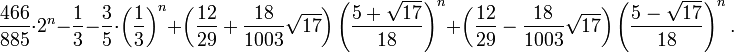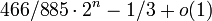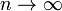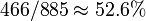## General Shortest Paths

A curious generalization of the original goal of the puzzle is to start from a given configuration of the disks where all disks are not necessarily on the same peg, and to arrive in a minimal number of moves at another given configuration. In general it can be quite difficult to compute a shortest sequence of moves to solve this problem. A solution was proposed by Andreas Hinz, and is based on the observation that in a shortest sequence of moves, the largest disk that needs to be moved (obviously one may ignore all of the largest disks that will occupy the same peg in both the initial and final configurations) will move either exactly once or exactly twice.

The mathematics related to this generalized problem becomes even more interesting when one considers the average number of moves in a shortest sequence of moves between two initial and final disk configurations that are chosen at random. Hinz and Chan Hat-Tung independently discovered that the average number of moves in an n-disk Tower is given by the following exact formula:Note that for large enough n, only the first and second terms are not converging to zero, so we get an asymptotic expression:, asThus, intuitively we could interpret the fraction ofas representing the ratio of the labor one has to perform when going from a randomly chosen configuration to another randomly chosen configuration, relative to the difficulty of having to cross the "most difficult" path of lengthwhich involves moving all the disks from one peg to another. An alternative explanation for the appearance of the constant 466/885 , as well as a new and somewhat improved algorithm for computing the shortest path, was given by Romik.